II.1.21cde

1/14/2021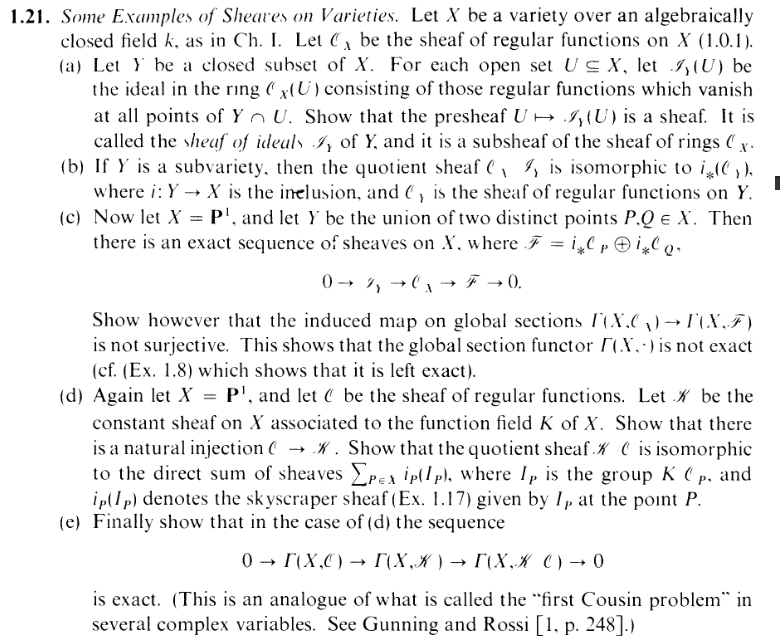AWWW, YES. We're finally relating what we've learned here a bit back to chapter 1. EXCITING! Get ready for some EBIC NOSTALGIABAIT! But first,

A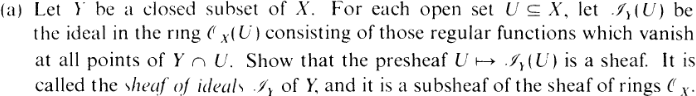>Verify that this presheaf is a sheaf BOOOOOOOOOOOOOOOOOOOOOOOOOOOOOOOORING. Skipping this one. It's NOT that I can't do it. It's just NOT WORTH IT. Bleh.

B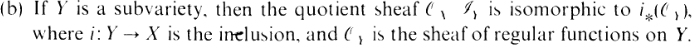Got stuck lol [Might add more detail later].

C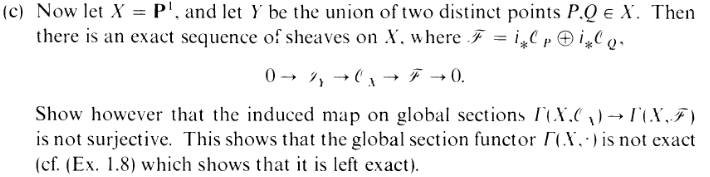Okay, FINALLY here's one I did. We wanna show the exactness of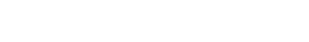(1)

which, as usual, we'll do at the stalk level. But first let's make these maps... Errr... Actually by definition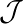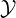is a subsheaf of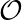X, so ϕ can just be the inclusion. For ψ on the other hand, we need to, given an open set U, define

 ψ(U) :X(U) →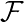(U)

Okay. Let's go ahead and unwrap the RHS:(U) = i*P(U) ⊕ i*Q(U) =

Okay lol, so before we move on, what does i*P look like? I mean, first of all, the regular functions on a singleton are necessarily just gonna be constant functions. So if P U, then it just becomes k. Otherwise U ∩{P} = , yielding a 0... Wait a second....

WHAT'S GOING ON?

ARGH! I THINK I'M GETTING A BIT OFHIGHER ON THE SHEAVES. AND I KNOW IT'S MY TIME TO GO.

uhh yea, so basically my point here is that i*X is a skyscraper sheaf on the point P with the constant ring k. AIN'T THAT SOMETHING? So depending on whether P and Q are in U,

 i*P(U) ⊕ i*Q(U) = (k or 0) × (k or 0)

(× is the same as for finite products of groups) AND THUS, the natural map for ψ(U) is just plain ol' evaluation

 f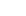f(P) × f(Q) (taking f(L) = 0 if f not defined at L)

Okay, now let's drop down to the stalk level and get exactness there: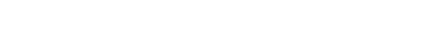(2)

Now, erm... I'm just gonna assume the direct product commutes over stalks... EXERCISE LEFT 2 READER:R = (i*P(U) ⊕ i*Q(U))R = (i*P(U))R ⊕ (i*Q(U))R

AWW YEA, MULTISTALK DRIFTING. And thanks to our work in 1.17, I know that these stalks are just k or 0, depend on R's inclusion in the closures of P and Q.... HOWEVER.... Recall this is X = P1, in which singletons are already closed!! So really, this depends on whether R is P or Q. And since P and Q are distinct...R =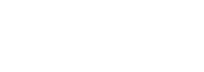Either way, ψP is clearly surjective (trivial forR = 0 and the constant functions coverR = k). So now all we have to verify for exactness of (2) is...

 imϕR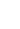ker ψR

Which I'll split into cases.

0.1 CASE: R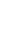{P,Q}

ThenR = 0 and ker ψR = (X)R. Oh, probably a good time to mention now that by virtue of ϕP being an injection, we can identify imϕP = (Y )R. OKAY. Now since S = {P,Q} is closed, we can obtain a neighborhood V or R that does not intersect S. Then CLEARLY,Y (V ) =X(V )

(since the restrict to 0 condition is vacuously true), and the same for all smaller neighborhoods of R, hence yielding

 (Y )R = (X)R

i.e. imϕR = ker ψR. Done

0.2 CASE: R = P or Q

Assume wlg R = P. ThenP = k, and

 ker ψP = {< V,f >∈X|f(P) = 0}

Which if ya think about it, is basically just a description of ()P. So we're done, haha.

0.3 SURJECTIVE BUT NOT SURJECTIVE

So here's an example of the famous OOPSIE that we've kept run into about surjectivity. We gotta show that the map of global sections

 ψ(X) :X(X) →(X)

is NOT surjective (despite ψ being surjective as a whole). Actually, this is ez. Since both P and Q are in X, I know that(X) = k ×k. And a theorem I.3.4 all the way back from Chapter I tells us thatX(X) = k. So our map is

 ψ(X) : k → k × k

where, following the definition of ψ, the image of ψ is the diagonal, a proper subset of k × k. DONE.

D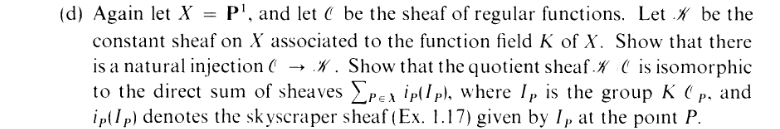Okay, first thing's first, let's go ahead and make the injection

This becomes obvious when you work out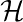. Going by the definition of constant sheaf, we can write(U) = {f : U → K|f continuous} (3)

Alright, so I fucked this up a couple of times confused as to what was going on... but then I realized something. Remember, the constant sheaf gives K a discrete topology, right? I also I know that if f(U), it has to be continuous. Now let's say s K is in the image of f (there is at least one such element). Now take another element t K. By continuity, f-1(s) and f-1(t) have to both be open. By assumption, f-1(s) is nonempty, but what about f-1(t). If it's NOT empty, then it intersects f-1(s), because open sets here are dense. But if st, we get a contradiction (f has points that map to two different things), so we must have s = t. So basically, all f does is pick out a point of K... so it can just be taken as an element of K. So (for nonempty U) I can just rewrte (3) as(U) = K

LMFAO. What a simplification. Also note, given open U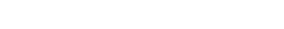(was unsure about that last equality at first, but it's thanks to good ol'birational equivalence)

Wee, so that's our injection.

And now we have to deal with this business about... what's that? Skyscraper sheaves?

THAT AWFUL PAIN

WE. ARE.IS A NEW WAY I LIKE TO BE.
I'M. JUST. RUNNING. IN. THE. 90S.

Okay, so we wanna show that∕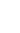∑ P∈XiP(K∕P) (4)

Okay, this looks scary, Let's plug in an open U X and see what happens.(U)∕(U)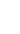∑ P∈XiP(K∕P)(U) i.e.K(U)∕(U)∑ P∈UK∕P

OHHHHHHHH BOY. This is some WILD stuff, lol. So sending an element from LHS RHS is evidently extremely trivial, but the reverse direction? We have to match up tons of copies of K to a SINGLE element of K, and our only hope is taking advantage of our quotient.

WHAT. THE. FUCK. I need to "glue" a bunch of K∕P elements into a SINGLE K(U)(U) element? This honestly doesn't even seem remotely possible because there's no restriction on the selections of each K∕P component. Yes, we are actually trying to prove that you can literally just pick a ton of random elements from K, and somehow when you quotient all of them byPs, it glues together into something.

Alright: For the first time in Hartshorned II so far, I am going to look up a solution. Maybe I should make a counter for how many times I do this (update: it's now on the list page)

UPDATE: I'M RETARDED.

I SOMEHOW forgot to try checking this at the stalk level. The mantra of sheaves has been "stalks are nice" and I FORGOT?!!?!:!?!? HOW?????? I know why: Because I was so used to going down to stalks when it came to the question of exactness; I forgot it would also be useful for the question of isomorphism. Let's start from (4) and try this again.∕∑ P∈XiP(K∕P) i.e. (∕)Q(∑ P∈XiP(K∕P))Q i.e.Q∕Q∑ P∈X(iP(K∕P))Q i.e. K∕Q(iP(K∕Q))Q i.e. K∕QK∕Q (from our work on skyscrapers)

AND THUS THAT QUESTIoN MARK IS NO MORE. DONE. HOW CONCRETELY THEY ACTUALLY MANAGE TO GLUE TOGETHER IDK, BUT THE STALKS TELL ME IT'S TRUE, THEREFORE IT'S TRUE. God bless stalks.

CUNNY IN THE 90S
IS A NEW WAY I LIKE /tv/
CUTE. AND. FUNNY IN THE 90S
COME ON CHILD RUN TO ME
WE. LOVE. CUNNY IN THE 90S
IS A NUDE WAY TO SET ME FREE
IT'S. JUST. CUNNY IN THE 90S
YES I WANNA UOHHHHH
YES I WANNA SEE

Note: holeinmyheart is now canceled.

E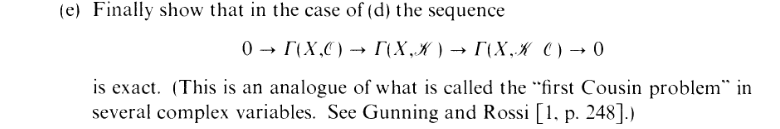Personally, I have another "first Cousin problem."

Note: holeinmyheart is now double-canceled. It's not clear what he was thinking here, but for some reason he decided to double down in order to perform the art of multi-cancel drifting, thus ending his internet career. He is finally free.

WHEN THE SUN GOES DOWN,
WILL YOU HEAR THE CRY,
OR JUST WALK ON BY,
AND LEAVE WITHOUT A SOUND.

Okay, offensive jokes aside, idiot Harshorne brought up the "first Cousin problem" way too late. He should have brought it up during part D, because that's more akin to the to what it actually is. Although I'm still having trouble "matching" the complex analysis version to our alggeo version. WHATEVER.

Well, anyway, if we rewrite their sequence in more familiar notation, we get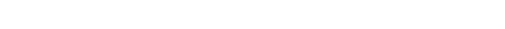And uhhh.... WE'RE DONE, LOL. Thanks to part D,(U) is a subgroup of(X), so this is just the first isomorphism theorem... In fact, does the sections even have to be global? I can replace X with open U in X and it would still work, wouldn't it? Guess that's it.

Next exercise is the last one of this section. Hartshorned II is almost at its first season finale. Yayyyyy!!! Also shoutout to cyuucat for the tip on how2glowtext. (Although I'm sure they disavow my use of it)